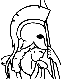#Network Flows and Monotropic Optimization Table of Contents:

1. Networks

1. Definition of a Network
2. Examples of Networks
3. Incidences
4. Flows
5. Divergence
6. Vector Operations
7. Circulations and the Augmented Network
8. Dynamic Version of a Network
9. Potentials and Tension
10. Preview of Optimal Flows and Potentials
11. Some Generalizations
12. Exercises

2. Paths and Cuts

1. Paths
2. Incidences for Paths
3. Connectedness
4. Finding a Path from One Place to Another
5. Cuts
6. Painted Network Algorithm
7. Priority Rules and Multiroutings
8. Theoretical Implications of the Algorithm
9. Application to Connectedness
10. Acyclic Networks
11. Planar Networks and Duality
12. Exercises

3. Flows and Capacities

1. Capacity Intervals
2. Flux across a Cut
3. Max Flow Problem
4. Max Flow Min Cut
5. Nature of the Min Cut Problem
6. Max Flow Algorithm
7. Commensurability and Termination
8. Feasible Flows
9. Feasible Distribution Algorithm
10. Multipath Implementation
11. Flow Rectification Algorithm
12. Node Capacities and Dynamic Flows
13. Exercises

4. Analysis of Flow

1. Integral Flows
2. Conformal Realization of Flows
3. Realization Algorithm
4. Justification of the Algorithm
5. Trees
6. Forrests and Spanning Trees
7. Tucker Representations of the Circulation Space
8. Basic Theorem
9. Pivoting
10. Extreme Flows
11. Extremal Representation Algorithm
12. Exercises

5. Matching Theory and Assignment Problems

6. Matching Problem
7. Matching Algorithm
8. Assignments
9. Application to Partially Ordered Sets
10. Optimal Assignments
11. Hungarian Assignment Algorithm
12. Matching with Multiplicities
13. Bottleneck Optimization
14. Exercises

• Potentials and Spans

1. Spread Relative to a Path
2. Optimal Paths
3. Max Tension Min Path
4. Min Path Algorithm
5. Node-Scanning Implementation
6. Feasible Potentials
7. Feasible Differential Theorem
8. Refinements
9. Tension Rectification Algorithm
10. Optimal Routings
11. Routing Optimization Procedure
12. Integral and Extreme Differentials
13. Exercises

• Networks with Linear Costs

1. Linear Optimal Distribution Problem
2. Examples of Optimization of Flows
3. Optimal Distribution Algorithm
4. Simplex Method for Flows
5. Linear Optimal Differential Problem
6. Examples of Optimization of Potentials
7. Optimal Differential Algorithm
8. Simplex Algorithm for Potentials
9. Duality and the Elementary Problems
11. Interpretations
12. Multipath Implementation
13. Out-of-Kilter Algorithm
14. Exercises

• Optimal Flows and Potentials

1. Convex Cost Functions
2. Characteristic Curves
3. Piecewise Linear or Quadratic Costs
4. Optimal Distribution Problem
5. Conjugate Costs
6. Examples of Conjugate Functions
7. Optimal Differential Problem
8. Duality Theorem and Equilibrium Conditions
9. Equilibrium Models
10. Improvement of Flows
11. Improvement of Potentials
12. Existence of Solutions
13. Boundeness of Optimizing Sequences
14. Black Boxes
15. Exercises

• Algorithms for Convex Costs

1. Optimal Distribution Algorithm
2. Application to Piecewise Linear Problems
3. Optimal Differential Algorithm
4. Thrifty Adjustment Algorithm (Piecewise Linear)
5. Application to Black Boxes
6. Out-of-Kilter Algorithm (Piecewise Linear)
7. Termination and Refinements
8. Fortified Algorithms and the Duality Theorem
9. Discretized Descent Algorithms
10. Calculating epsilon-Optimal Solutions
11. Optimizing Sequences and Piecewise Linearization
12. Convex Simplex Method
13. Exercises

• Linear Systems of Variables

1. Primal and Dual Variables
2. Elementary Vectors and Supports
3. Bases
4. Networks with Gains
5. A Generalization of Circuits and Cuts
6. Multicommodity Systems and Factorization
7. Painted Index Theorem and Algorithm
8. Termination and Preprocessing
9. Constraints and Feasibility
10. Rectification Algorithms
11. Shortcuts in Pivoting Implementation
12. Augmented and Aggregated Formats
13. Extreme Solutions
14. Exercises

• Monotropic Programming

1. Optimization and Equilibrium
2. Examples of Monotropic Programming
3. Descent by Elementary Vectors
4. Duality and the Existence of Solutions
5. Boundedness Property
6. Decomposition
7. Application to Traffic Equilibrium
8. Basic Descent Algorithms
9. Fortified and Discretized Descent
10. Simplex Methods
11. General Out-of-Kilter Algorithm
12. Other Formats and Termination
13. Parametric Programming
14. Exercises